## Iterated complex factorial. Gamma function fractals ¶

Recently we came across a discussion on Gamma Function and Fractal Factorials, having as starting point this blogpost.

In this IPython Notebook we give the Python code to reproduce the Mathistopheles' results, and experiment further with this kind of fractals.

The general properties of Gamma function can be found here.

The basic idea is to study through numerical experiments how behaves the discrete dynamical system defined by the complex function $f(z)=\Gamma(z+1)$.

To study the dynamics of this map, one computes iterates $f^n=f\circ f \circ \cdots \circ f$, and the orbit of a point $z$ through $f$ is the sequence $(z_n)$ with $z_n=f^n(z)$.

Since for positive integers we have $\Gamma(n+1)=n!$, one extends the factorial to a complex number $z$, $z\neq k$, with $k$ an integer, $k\leq 0$. Namely $z!=\Gamma(z+1)=f(z)$. The iterated factorial of $z$ is $f^n(z)$. For example $((z!)!)!=f^3(z)$.

We start with a numerical experiment illustrating dynamical behaviour of a few orbits.

In :
from scipy.special import gamma
import numpy as np
import matplotlib.pyplot as plt
from mpl_toolkits.axes_grid1 import make_axes_locatable # we need this function to locate colorbars
%matplotlib inline


Let us compute a segment of orbit of a point $z_0=z$, i.e. a few terms of the associated sequence $z_n=\Gamma(z_{n-1}+1)$:

In :
z=1+2*1j
for k in range(25):
z=gamma(z+1)
print  z

(0.112294242346326 + 0.323612885501927j)
(0.874251610757277 - 0.106907952790729j)
(0.948777931190684 - 0.0344226143472293j)
(0.978939780955043 - 0.0131190526063302j)
(0.991207960208101 - 0.00532017265947946j)
(0.996303046707114 - 0.00221084553826231j)
(0.998440602150385 - 0.000927985087495601j)
(0.999341357858007 - 0.000391146097995793j)
(0.999721652071882 - 0.000165158278610297j)
(0.999882339530896 - 6.97884699652631e-5j)
(0.999950258692482 - 2.94987085551536e-5j)
(0.999978970814976 - 1.2470383308318e-5j)
(0.999991109308073 - 5.27206671295952e-6j)
(0.999996241175832 - 2.22890861209908e-6j)
(0.999998410831796 - 9.42340744709546e-7j)
(0.999999328125252 - 3.98405671695687e-7j)
(0.999999715942002 - 1.68439456525581e-7j)
(0.99999987990475 - 7.12135242212195e-8j)
(0.999999949225613 - 3.01079554434357e-8j)
(0.999999978533385 - 1.27291706641559e-8j)
(0.999999990924252 - 5.38169373052753e-9j)
(0.999999996162916 - 2.27529576533372e-9j)
(0.999999998377741 - 9.6195940010783e-10j)
(0.999999999314134 - 4.06701364080917e-10j)
(0.999999999710027 - 1.7194696556683e-10j)


We notice that the sequence $z_n$ approaches 1.

In :
z=3-0.2*1j
for k in range(10):
z=gamma(z+1)
print  z

(5.77861687872-1.48371352226j)
(-369.648653382-156.601091536j)
(-0-0j)
(1+0j)
(1+0j)
(1+0j)
(1+0j)
(1+0j)
(1+0j)
(1+0j)
(1+0j)
(1+0j)
(1+0j)
(1+0j)
(1+0j)

In :
z=2.9899809160305342-0.18574297188755021*1j
for k in range(10):
z=gamma(z+1)
print  z

(5.73689190891-1.36064649141j)
(-306.495252172-227.687734931j)
(nan+nan*j)
(nan+nan*j)
(nan+nan*j)
(nan+nan*j)
(nan+nan*j)
(nan+nan*j)
(nan+nan*j)
(nan+nan*j)


Choosing randomly points in the complex plane, their orbits either approach 1 or after a number of iterations the function gamma returns a nan (not a number), except for fixed points of the function $f(z)=\Gamma(z+1)$, i.e. points $z$, sucha that $f(z)=z$.

$z=1$ and $z=2$ are fixed points:

Let us check if $z_0=1$ and $z_0=2$ are attracting, repelling or neutral fixed points, i.e. if $|f'(z_0)|$ is less than 1, greater than 1 or equal to 1. For we import mpmath library:

In :
import mpmath as mpm


mpmath is a Python library for real and complex floating-point arithmetic with arbitrary precision. It provides functions to evaluate special mathematical functions such as gamma, zeta, hurwitz, and more. The function of interest for gamma fractals is mpmath.factorial(z).

The derivative of mpm.factorial at 1 is:

In :
deriv = mpm.diffs(mpm.factorial, 1) # deriv is a generator
print next(deriv)

1.0

In :
deriv = mpm.diffs(mpm.factorial, 2)
print next(deriv)

2.0


Hence $1$ is a neutral fixed point and 2 is repelling. Since $f'(1)=1$ is a root of unity, $1$ is a rationally neutral fixed point.

Open question: Does this function exhibit more fixed points or periodic points (points $z$ such that there exists a positive integer $q$, and $f^q(z)=z$)?

In order to illustrate how fast the orbits are attracted by 1 or they reach a not a number, nan, we adapt to this case the Escape Time Algorithm used to generate Julia sets.

To each point $z_0$ one associates an integer $n$, which is either the first iterate that is at a distance less than $0.005$ from $1$ or the modulus $|z_n|$ is a nan. If in a prescribed number of iterations, Miter, no one of the two conditions is met than the Miter is associated to that point:

In :
def iterGamma(z,Miter):

for n in range(Miter):
if  np.abs(z-1)<0.005  or np.isnan(np.abs(z)):
return n
z=gamma(z+1)

return Miter


The next Python function generates the visual representation of the patterns associated to the function $f(z)=\Gamma(z+1)$, through the above algorithm. More precisely, to a narrow grid of a rectangular region in the complex plane, one associates an array w of integer numbers, n, returned by the iteratedGamma function. This array is transformed into an image, mapping the n values to a colormap.

After many experiments we decided to use the cubehelix colormap for most of our plots in this IPython Notebook. The list of all colormaps provided by matplotlib can be found here.

In :
def plotGammaiterated(re, im,  cmap, filename=False, N=100, Miter=50):

# re is a tuple giving the interval on the real axis for the rectangular region
# im is a tuple giving the interval on the imaginary axis
# cmap is the name of the colormap used to plot the fractal set
# filename is a string such as 'imagefile.png'
# N is the number of points in an unit interval

Nx=int((re-re)*N)# horizontal resolution
Ny=int((im-im)*N)# vertical resolution
x=np.linspace(re, re, Nx)
y=np.linspace(im, im, Ny)
w=np.zeros((Ny,Nx), dtype=int)

for n in xrange(Ny):
for m in xrange(Nx):
z =x[m]+1j*y[n]
w[n][m] = iterGamma(z, Miter)

fig=plt.figure()

im=plt.imshow(w, cmap=cmap, extent=(re, re, im, im), interpolation='nearest', origin='lower')
divider = make_axes_locatable(ax)
plt.colorbar(im, cax=cax)

if (filename):
plt.savefig(filename)

In :
plt.rcParams['figure.figsize']=11,  12

In :
plotGammaiterated((-5.5, 0),(-2,2), 'cubehelix' )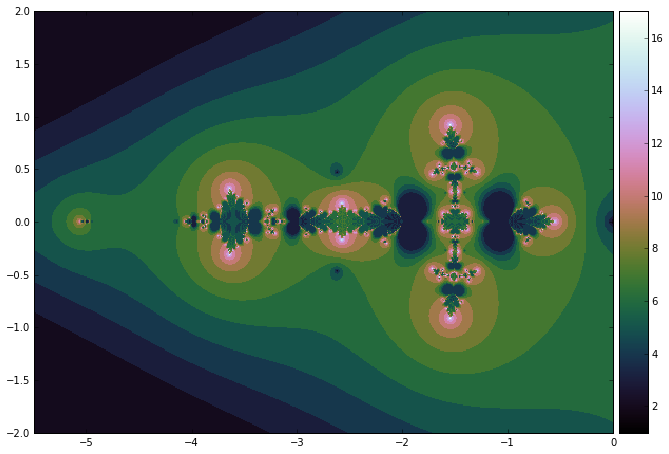Enlarging the above rectangle we get:

In :
plotGammaiterated((-5, 5),(-5,5), 'cubehelix' )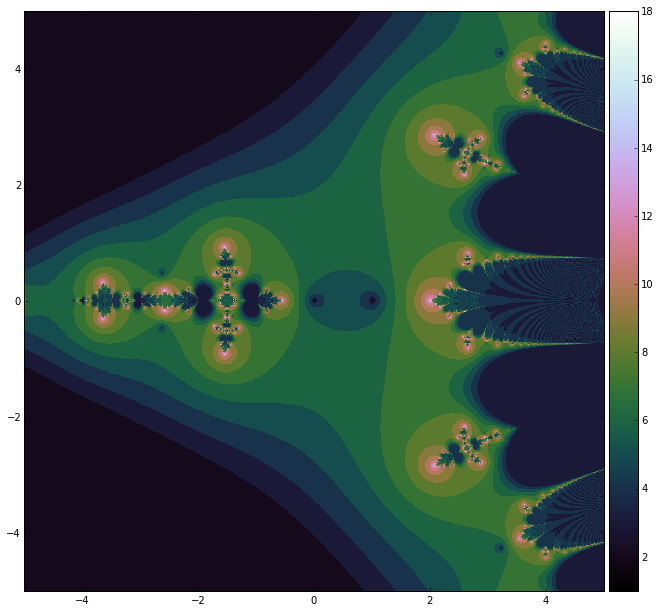We change the colormap:

In :
plotGammaiterated((-5, 5),(-5,5), 'gist_earth' )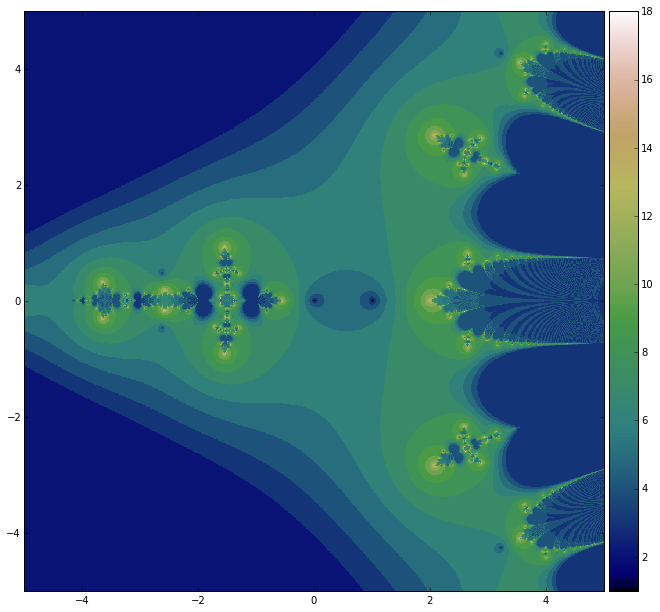Now let us zoom a subregion in the above plot:

In :
plt.rcParams['figure.figsize']=9,9

In :
plotGammaiterated((1.75, 3.75),(2,3.2), 'cubehelix', N=300, filename='gammafr.png' )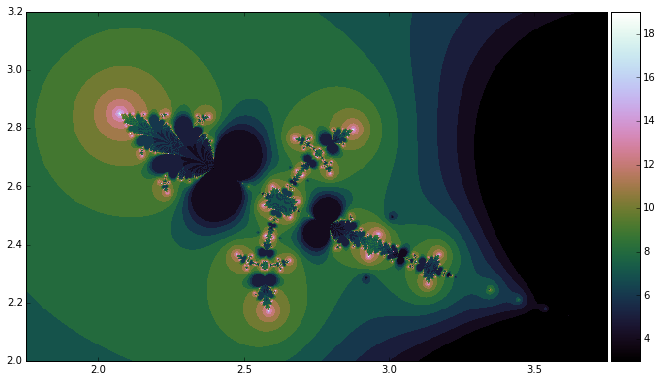In order to test the conjecture that the orbits of some points approach the fixed point 1, while other escape to infinity or none of these conditions is met, we define a new function that returns a tuple of four elements, $(r,g,b,a)$, representing respectively the colors yellow, black and white.

• yellow encodes the points whose orbit approaches 1
• black encodes the points whose iterates escape to infinity
• white encodes the third type of points (fixed points and possible points in other $f$-invariant sets).
In :
def iterGamma3cols(z, Miter):

for n in range(Miter):
if  np.abs(z-1)<0.005:
return   (1.0, 0.8,  0.0, 1.0)# yellow

if  np.isnan(np.abs(z)):
return (0,0,0,1) #black
z=gamma(z+1)

return (1,1,1,1) #white

In :
def plotGammaiter3cols(re, im,   N=100, Miter=50, filename=False):

Nx=int((re-re)*N)
Ny=int((im-im)*N)
x=np.linspace(re, re, Nx)
y=np.linspace(im, im, Ny)
w=np.zeros((Ny, Nx, 4), dtype=float)

for n in xrange(Ny):
for m in xrange(Nx):
z =x[m]+1j*y[n]
w[n][m] = iterGamma3cols(z, Miter)

fig=plt.figure()

im=plt.imshow(w,  extent=(re, re, im, im), interpolation='nearest', origin='lower')

if(filename):
plt.savefig(filename)

In :
plt.rcParams['figure.figsize']=10,10

In :
plotGammaiter3cols(re=(1,7), im=(-6,6))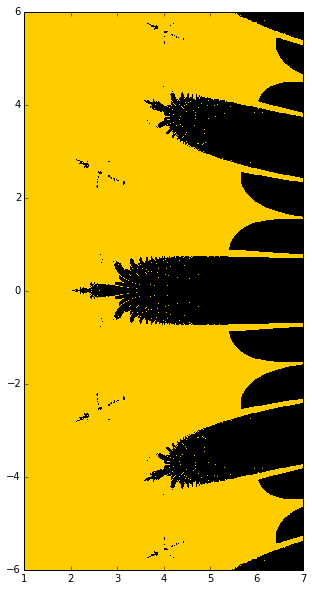Let us zoom the central protuberance:

In :
plt.rcParams['figure.figsize']=10, 6

In :
plotGammaiter3cols(re=(1.75,6.5), im=(-1,1))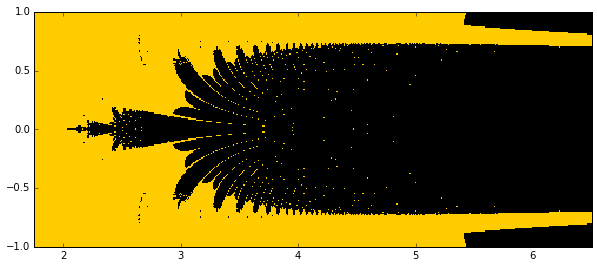The colored representation of this protuberance is the following:

In :
plotGammaiterated((1.75,6.5), (-1,1), 'cubehelix', N=300, Miter=100)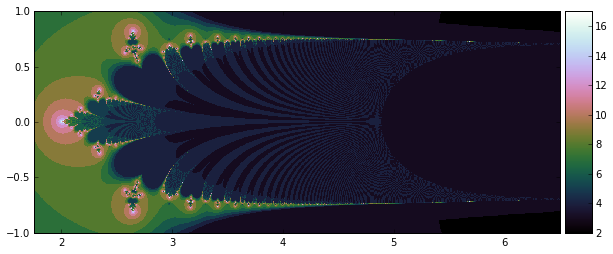A new zoom:

In :
plotGammaiter3cols(re=(1.95,3), im=(-0.25,0.25), N=500)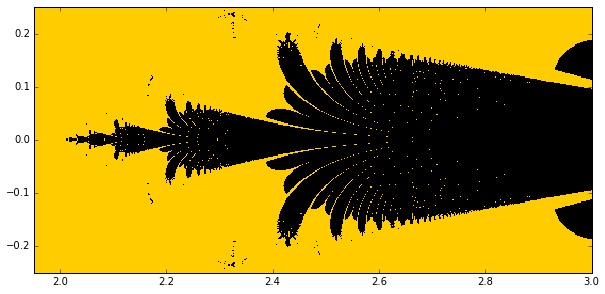and its colored version illustrates the self similarity:

In :
plotGammaiterated((1.95,3), (-0.25,0.25), 'cubehelix', N=500, Miter=100)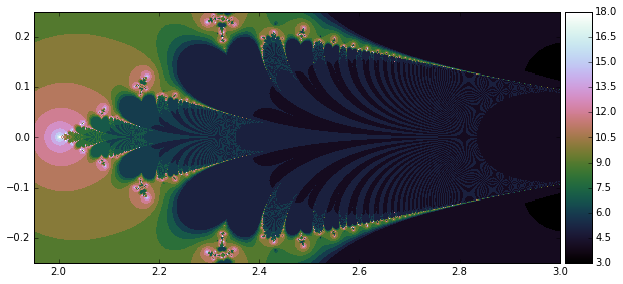In :
plt.rcParams['figure.figsize']=8, 8

In :
plotGammaiter3cols(re=(-4.5,0), im=(-1.25,1.25), N=200)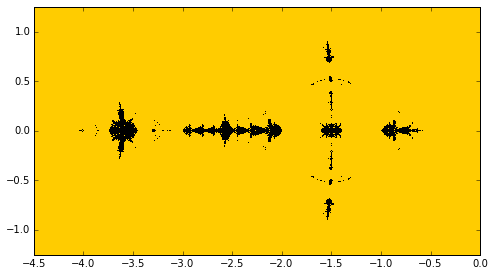In :
plotGammaiterated((-4.5,0), (-1.25,1.25), 'cubehelix', N=200, Miter=100)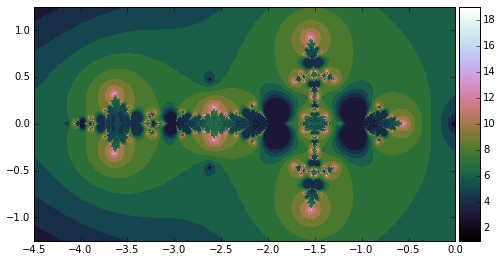To be continued :)

This IPython Notebook is included in the folder Math: https://github.com/empet/Math/blob/master/README.md

In :
from IPython.core.display import HTML
def  css_styling():